## Challenge

You are given a server script

``````import numpy as np
import sys
import glob
import string
import tensorflow as tf
from keras.models import Sequential
from keras.layers.core import Dense, Flatten
from flag import flag
import signal

signal.alarm(120)

tf.compat.v1.disable_eager_execution()

image_data = []
for f in sorted(glob.glob("images/*.png")):
f, grayscale=False, color_mode="rgb", target_size=None, interpolation="nearest"
)
im = tf.keras.preprocessing.image.img_to_array(im)
im = im.astype("float32") / 255
image_data.append(im)

image_data = np.array(image_data, "float32")

# The network is pretty tiny, as it has to run on a potato.
model = Sequential()
# I'm sure we can compress it all down to four numbers.

print("Train this neural network so it can read text!")

wt = model.get_weights()
while True:
print("0. Clear weights")
print("1. Set weight")
print("3. Quit")
inp = int(input())
if inp == 0:
wt.fill(0)
wt.fill(0)
wt.fill(0)
wt.fill(0)
model.set_weights(wt)
elif inp == 1:
print("Type layer index, weight index(es), and weight value:")
inp = input().split()
value = float(inp[-1])
idx = [int(c) for c in inp[:-1]]
wt[idx][tuple(idx[1:])] = value
model.set_weights(wt)
elif inp == 2:
results = model.predict(image_data)
s = ""
for row in results:
k = "?"
for i, v in enumerate(row):
if v > 0.5:
k = chr(i)
s += k
print("The neural network sees:", repr(s))
if s == flag:
print("And that is the correct flag!")
else:
break
``````

That describes building a sequential neural network

``````model = Sequential()
``````

This is a simple 2 layer classifier network with a bottleneck of 4.

As well as some 4 example images of 16x16 px RGB, that aren’t too relevant for the solution and just useful for testing.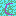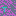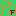This script allows the user to set the weights (even index) and biases (odd index) of a neural network

0) All to zero 1) To a chosen (integer) value

As well as

2) Running all images through the classifier and outputting a class label of 0-127 as an ascii value.

## Solution approach

To do some simple mathematics, we need to establish some variables.

The network has 4 weight parameters with the following shapes

``````# Dense(4, activation='relu')
w0: input x 4  # the weight matrix that's dot-product'ed with the input of the input
w1: 4  # the bias matrix that's added afterwards
# Dense(128, activation='softmax')
w2: 4 x 128  # the weight matrix that's dot-product'ed with the input of the previous layer
w3: 128  # the bias vector that's added afterwards
# Output vectors
o1: 4
o2: 128
``````

The calculation (ignoring activation functions) therefore is: ( @ = dot product, in numpy)

``````o1 = input @ w0 + w1
# relu on o1
o2 = o1 @ w2 + w3
# softmax on o2
``````

Assuming an otherwise empty matrix, just with the 0th element of each set, we can simplify this to simple scalars:

``````o1 = input[input_idx] * w0[input_idx, 0] + w1  # assuming the rest of the matrix is set to zero, nothing more is added here
o2[output_index] = o1 * w2[0, output_index] # + w3[output_index], this part isn't actually needed
``````

In the empty-matrix-assumption, all other o2 are 0, therefore the softmax will be 1 at output_index and 0 elsewhere. If we set the output_index to a printable character, i.e. ‘0’ == 48, it will print “0” if the o2[output_index] is > 0.

By setting the weight `w0[input_idx, 0]` to -255 and the bias `w1` to some threshold, we get `o1 = input[input_idx] * -255 + treshold`. This leads to a positive number, if the input (scaled to 0-255) is bigger than the threshold and a negative number otherwise. As the layer has a relu function, the negative values will be clippd to 0.

So thanks to the relu-function, we can construct a function that’s

``````0 if input[input_idx] > treshold
>0 if input[input_idx] < treshold
``````

We simply pass the output of `o1` to the `o2[output_index]` by setting the `w2[0, output_index]` to 1, and therefore completing our neural network oracle that gives us:

``````'a' if input[input_idx] > treshold
'?' otherwise
``````

To find the exact value of each pixel, we’d have to run this 256 times per pixel-channel, so 256*768 times, but luckily only knowing the 2 most significant bits of the color are enough to see the flag.

## Solution - local - code

First we import some stuff and read the data like in the initial script, as well as a function to display a stack of images.

``````import glob
import subprocess

import matplotlib.pyplot as plt
import numpy as np
import pwn
import tensorflow as tf
from keras.layers.core import Dense, Flatten
from keras.models import Sequential
from tqdm import tqdm

tf.compat.v1.disable_eager_execution()

image_data = []
for f in sorted(glob.glob("images/*.png")):
f, grayscale=False, color_mode="rgb", target_size=None, interpolation="nearest"
)
im = tf.keras.preprocessing.image.img_to_array(im)
im = im.astype("float32") / 255
image_data.append(im)
image_data = np.array(image_data, "float32")

def show(res):
for r in [img.reshape(16, 16, 3) for img in res.T.astype(int)]:
plt.imshow(r)
plt.show()
``````

Then we create a Simulator-class

``````class Simulator:
def __init__(self, image_data):
self.model = Sequential()
self.outpos = ord("0")
self.image_data = image_data
self.size = 4

def set(self, layer, pos, val):
wt = self.model.get_weights()
wt[layer][pos] = val
self.model.set_weights(wt)

def set_zero(self):
wt = self.model.get_weights()
for w in wt:
w.fill(0)
self.model.set_weights(wt)

def set_w0(self, pxpos, val=-255):
self.set(0, (pxpos, 0), val)

def set_b0(self, tresh):
self.set(1, 0, tresh)

def set_w1(self):
self.set(2, (0, self.outpos), 1)

def predict(self, pxpos, tresh):
pred = self.model.predict(image_data)
return pred[:, self.outpos] > 0.5

def run(self, steps=2):
res = np.zeros((768, self.size))  # array to store results
step_size = 256 // steps

self.set_zero()
self.set_w1()

tq = tqdm(range(0, 768))
for pxpos in tq:
self.set_w0(pxpos)
for tresh in range(step_size, 256, step_size):
self.set_b0(tresh)
r = self.predict(pxpos, tresh)
res[pxpos] += r * step_size
# tq.set_postfix(p=pxpos, v=res[pxpos])
self.set_w0(pxpos, val=0)
return res
``````

This class a) Creates an internal neural network like in the server b) Allows us to do the 3 critical set operations: Setting the w0, b0 and w1 for input-scaling, tresholding and output-mapping. c) Allows us to pass the data through the prediction d) Allows us to run over all pxpos (input pixel positions) as well as some tresholds, and writes it to a target result numpy array. The output-array has the 768 pixel-color-channels and space for oracle-information of 4 images.

With this script (given the images in the /images) folder, you are able to reconstruct the 2 MSBs of the original images in <10 seconds.

Calling the show, we get the following results: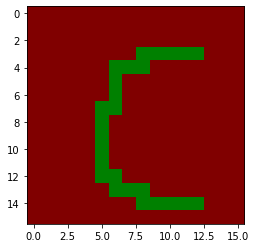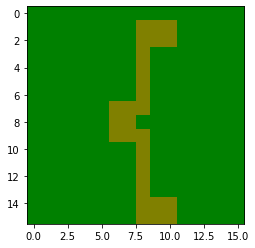## Simulator - Remote

To run this code on the server (or against or local server script for testing) we have to modify some simulator commands, by overriding some methods of the existing simulators to trigger the code on the server.

In the init-process we use pwntools to establish a connection to the script (local or remote) - and for the remote-case we solve the proof of work challenge.

Unlike our local script, we have to trigger the code from the menu according to the server logic:

``````    print("Menu:")
print("0. Clear weights")
print("1. Set weight")
print("3. Quit")
``````

So our modified remote simulator looks like this:

``````class PwnSimulator(Simulator):
def __init__(self, local):
super(PwnSimulator, self).__init__(None)
if local:
self.size = 4
self.proc = pwn.process(["/bin/python", "./server.py"])
else:
self.size = 34
self.proc = pwn.remote("ocr.2022.ctfcompetition.com", 1337)
chall = self.proc.recvuntil(b"===================").split(b"\n")[-2].strip().decode()
chall_response = subprocess.check_output(["sh", "-c", chall])
self.proc.writeline(chall_response)
print(f"Solved challenge {chall} with {chall_response}")

def set_zero(self):
self.proc.writeline("0")
self.proc.recvuntil(b"Quit", timeout=20)

def set(self, layer, pos, val):
self.proc.writeline("1")
try:
pos = " ".join(map(str, pos))
except:
pass
self.proc.writeline(f"{layer} {pos} {val}")
self.proc.recvuntil(b"Quit", timeout=1)

def predict(self, pxpos, tresh):
self.proc.writeline("2")
The resulting flag is `CTF{n0_l3aky_ReLU_y3t_5till_le4ky}`
A ipython notebook with all the code (and the flag as images) can be found